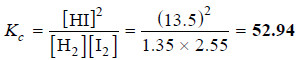# Calculation of Kc from Experimental Information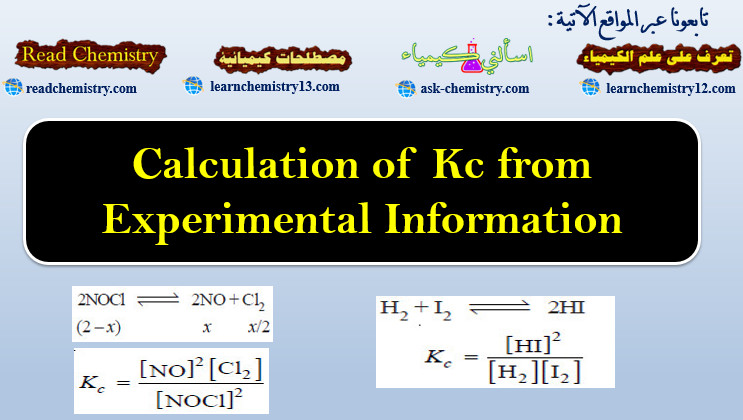## Calculation of Kc from Experimental Information

– To determine the value of Kc of a reaction, write the balanced equation. Then write the equilibrium constant expression.

– Substitute in it the equilibrium concentrations of the reactants and products found experimentally. Thus calculate the value of Kc.

### Solved problem (1) for Calculation of Kc

At equilibrium for the reaction:

2SO2(g) + O2 (g) ↔ 2SO3 (g)

the concentrations of reactants and products at 727°C were found to be: SO2 = 0.27 mol L–1; O2 = 0.40 mol L–1; and SO3 = 0.33 mol L-1. What is the value of the equilibrium constant, Kc, at this temperature?

Solution:

– Write the equilibrium constant expression for the reaction: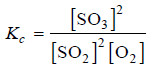– We know that:

[SO3] = 0.33 mol L–1; [SO2] = 0.27 mol L–1; [O2] = 0.40 mol L–1

– Substituting these values in the equilibrium constant expression, we have: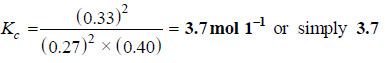### Solved problem (2)

Some nitrogen and hydrogen gases are pumped into an empty five litre glass bulb at 500°C. When equilibrium is established, 3.00 moles of N2, 2.10 moles of H2 and 0.298 mole of NH3 are found to be present. Find the value of Kc for the reaction.

N2(g) + 3H2(g) ↔ 2NH3(g)

at 500°C.

Solution:

– The equilibrium concentrations are obtained by dividing the number of moles of each reactant and product by the volume, 5.00 litres. Thus,

[N2] = 3.00 mole/5.00 L = 0.600 M

[H2] = 2.10 mole/5.00 L = 0.420 M

[NH3] = 0.298 mole/5.00 L = 0.0596 M

– Substituting these concentrations (not number of moles) in the equilibrium constant expression, we get the value of Kc.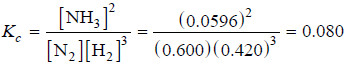– Thus, for the reaction of H2 and N2 to form NH3 at 500°C, we can write: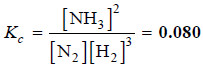### Solved problem (3)

Calculate the equilibrium constant at 25°C for the reaction:

2NOCl(g) ↔ 2NO(g) + Cl2 (g)

In an experiment, 2.00 moles of NOCl were placed in a one-litre flask and the concentration of NO after equilibrium achieved was 0.66 mole/litre.

Solution:

– Let us write the equilibrium constant expression for the balanced chemical equation.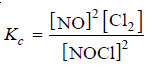– Next we proceed to find the equilibrium concentrations of NOCl, NO and Cl2.

– Since the volume of the reaction vessel is one litre, the moles of various reagents also represent their molar concentrations.

– The initial amount of NOCl is 2.00 moles.

– Let us assume that x moles of it have reacted to achieve the equilibrium.

– The moles of different reagents at equilibrium are indicated in the equation.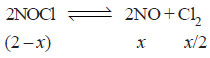– According to this equation, one mole of NOCl gives one mole of NO and half a mole of Cl2.

– The experimental value of equilibrium concentration of NO is 0.66 mole/litre.

– Therefore, the equilibrium concentration Cl2 is half of it i.e. 0.33 mole/litre. Since x = 0.66, the equilibrium concentration of NOCl = (2 – 0.66) = 1.34. To find Kc , these values of equilibrium concentrations are substituted in the equilibrium constant expression.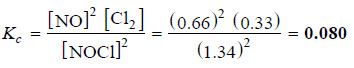### Solved problem (4)

At a certain temperature, 0.100 mole of H2 and 0.100 mole of I2 were placed in a one-litre flask. The purple colour of iodine vapour was used to monitor the reaction. After a time, the equilibrium

H2 + I2 ↔ 2HI

was established and it was found that the concentration of I2 decreased to 0.020 mole/litre.

Calculate the value of Kc for the reaction at the given temperature.

Solution:

– The equilibrium constant expression for the reaction: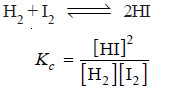– Let us proceed to find the equilibrium concentrations of the various species.

– The initial moles of H2 , I2 and HI present at equilibrium are: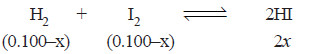moles at equilibrium

– Since the volume of the reaction vessel is one litre, the moles at equilibrium also represent the equilibrium concentrations. Thus,

[I2] = 0.100 – x = 0.020 (given)

or   x = 0.100 – 0.020

= 0.080

– Knowing the value of x, we can give the equilibrium concentrations of H2 , I2 and HI.

[H2] = 0.100 – x = 0.100 – 0.080 = 0.020

[I2] = 0.020 (given)

[HI] = 2x = 0.160

– Substituting these values in the equilibrium constant expression, we get the value of Kc.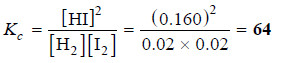### Solved problem (5)

13.5 ml of HI are produced by the interaction of 8.1 ml of hydrogen and 9.3 ml of iodine vapour at 444°C. Calculate the equilibrium constant at this temperature of the reaction.

H2(g) + I2 (g) ↔ 2HI (g)

Solution:

– The equilibrium constant expression for the reaction is: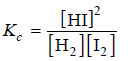– Let us proceed to find the equilibrium concentrations.

– Since the number of moles in a gas under the same conditions of temperature and pressure are proportional to volumes, ml of a gas may be used instead of molar concentrations. Thus,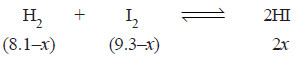– The experimental volume of HI = 13.5 ml (given)

∴ 2x = 13.5 ml

or   x = 6.75 ml

– Thus, the equilibrium concentrations are:

[H2] = (8.1 – x) = 8.1 – 6.75 = 1.35

[I2] = (9.3 – x) = 9.3 – 6.75 = 2.55

[HI] = 13.5

– Substituting these values in the equilibrium constant expression, we have: